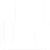## Typical Waveforms for the AV-1010-B with a Current-Boosting Transformer

The AV-1010-B pulse generator can generate up to ±100V into a 50Ω load, which represents a current of ±2 Amps. If a pulse transformer attached to the output, the output voltage can be lowered, and the output current boosted - to as much as 8 Amps. This technique is illustrated in the AV-1010-B datasheet, and Section H of Application Note 3A. This Technical Brief shows actual waveforms obtained by this technique.

Figure 1 shows a +100V pulse generated by the AV-1010-B directly into a 50Ω load. No transformers are used in this case, and the pulse generator output impedance is set at 2 Ohms.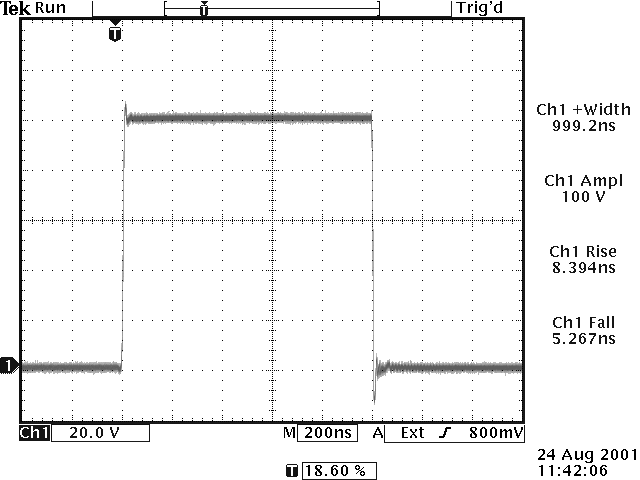Figure 1  AV-1010-B Output Directly into a 50 Ohm load

Figure 2 shows the output into a 3 Ohm load when the same pulse generator settings are used, but an AVX-MRB6 transformer is inserted between the load and the pulse generator. The AVX-MRB6 is connected to the AV-1010-B with a 24" length of RG58C/U coaxial cable, and the 3 Ohm load is soldered directly to the output of the AVX-MRB6. The AVX-MRB6 reduces the voltage by a factor of 4, and increases the current by a factor of 4. (The required load impedance is thus 50 Ω / 42 = 3.125 Ω). Some oscillations can be observed, due to an imperfect impedance match. These oscillations will be more pronounced if the length of cabling is longer, and conversely they will be less pronounced  if the cabling is shorter.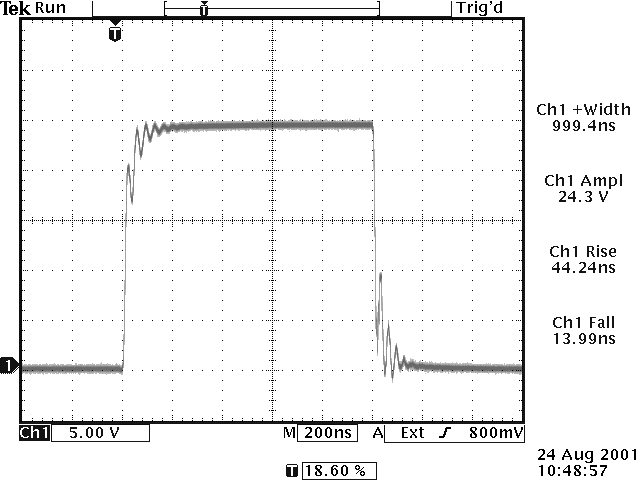Figure 2  AVX-MRB6 Output into a 3 Ohm load, with
the AV-1010-B Output Impedance set at 2 Ohms

By setting the pulse generator output impedance to 50Ω, these oscillations can be eliminated. This is because the 50Ω output impedance provides transmission line back-matching - that is, the output impedance absorbs the transmission line reflections. The drawback is that the output current is reduced by a factor of two, to 4 Amps. This is shown in Figure 3.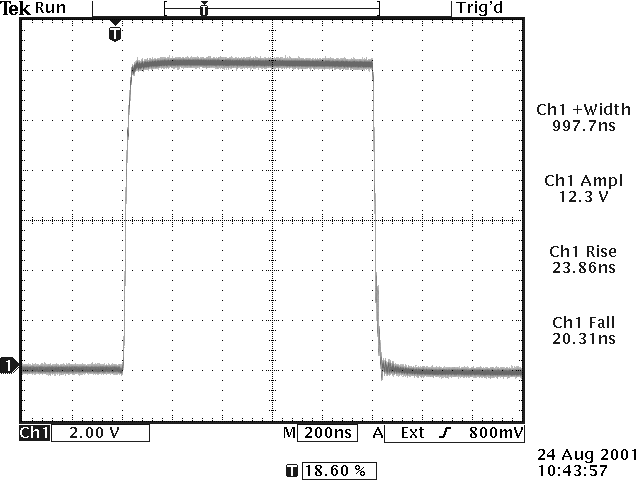Figure 3  AVX-MRB6 Output into a 3 Ohm load, with
the AV-1010-B Output Impedance set at 50 Ohms

The addition of the output transformer does degrade the rise and fall time somewhat.

Overall, the combination of the AV-1010-B pulse generator with an output transformer allows for a highly flexible test system, ideal for testing both 50 Ohm loads, laser diodes, and other devices.### info@avtechpulse.com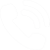### ph. 888-670-8729 or +1-613-686-6675